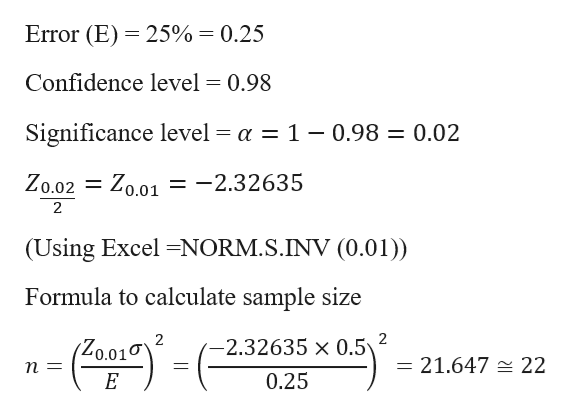The combined city/highway fuel economy of a 2016 Toyota 4Runner 2WD 6-cylinder 4-L automatic 5-speed using regular gas is a normally distributed random variable with a range 22 mpg to 25 mpg. (a) Estimate the standard deviation using Method 3 (the Empirical Rule for a normal distribution) from Table 8.11. (Round your answer to 4 decimal places.)  Standard deviation        _________      (b) What sample size is needed to estimate the mean with 98 percent confidence and an error of ± 0.25 mpg? (Enter your answer as a whole number (no decimals). Use a z-value taken to 3 decimal places in your calculations.)  Sample size            _________

Question

The combined city/highway fuel economy of a 2016 Toyota 4Runner 2WD 6-cylinder 4-L automatic 5-speed using regular gas is a normally distributed random variable with a range 22 mpg to 25 mpg.

(a) Estimate the standard deviation using Method 3 (the Empirical Rule for a normal distribution) from Table 8.11. (Round your answer to 4 decimal places.)

Standard deviation        _________

(b) What sample size is needed to estimate the mean with 98 percent confidence and an error of ± 0.25 mpg? (Enter your answer as a whole number (no decimals). Use a z-value taken to 3 decimal places in your calculations.)

Sample size            _________

Step 1

Given Data

Range from 22 mpg to 25 mpg

a = 22 mpg

b = 25 mpg

a) Standard Deviation using method 3 for normal distribution is calculated as

Step 2

b)

Estimation of sample size...help_outlineImage TranscriptioncloseError (E) 25% = 0.25 Confidence level = 0.98 Significance level = a = 1 - 0.98 = 0.02 Zo.02 Zo.01 = -2.32635 1 2 (Using Excel -NORM.S.INV (0.01)) Formula to calculate sample size 2 2 2.32635 x 0.5 Zo.01 = 21.647 = 22 1 0.25 E fullscreen

Want to see the full answer?

See Solution

Want to see this answer and more?

Our solutions are written by experts, many with advanced degrees, and available 24/7

See Solution
Tagged in

Other